## Loops, Splitting, and Operators

##### Learning

When constructing or running simulations, you may want to query or modify values associated with all, or some of, the objects in your model (such as zones, nodes, blocks, balls, contacts, rockbolts, etc.). This may be to measure results like stress or displacement, to assign a calculated extra variable for plotting, or to adjust a property value. There are several ways to identify and navigate across all these objects using loops, splitting, and operators — with each one becoming easier and faster to execute.

Since the earliest Itasca software, such objects were stored in a linked list. The standard approach was to use a While Loop to traverse all the pointers of any object.

Several versions ago, a new ForEach Loop statement was added to FISH to simplify iterating through all the different objects. This approach simplifies the FISH function, especially for 3DEC where you may need to traverse across all the blocks and zones within each block, requiring nested loops using the Loop While approach. Note that if you are using the “converto” tool in 3DEC or FLAC3D, any While Loops will be retained, although with updated syntax (e.g., zone_head will become zone.head), and not converted to ForEach Loops.

With the advent of multi-threaded FISH, splitting and operators became available. FISH splitting allows a function to be executed repeatedly on each element (object) of an aggregate type (a list, array, container of objects, etc.). FISH operators are a special class of function designed to be executed in a multi-threaded environment.

This tutorial concludes by reviewing the model property distributions via the PROPERTY command.

## Example

In the FLAC3D Hoek-Brown Slope example in the documentation, and shown below, a constant value of 30 MPa (highlighted in yellow) is assigned across the model for the Hoek-Brown intact rock strength parameter (σci) — the Hoek-Brown constitutive model property constant-sci — in FLAC3D. Note that the command model random 9999 has been added, where 9999 is the seed value. This ensures that the same pseudo-random values will be generated in the model each time it's run.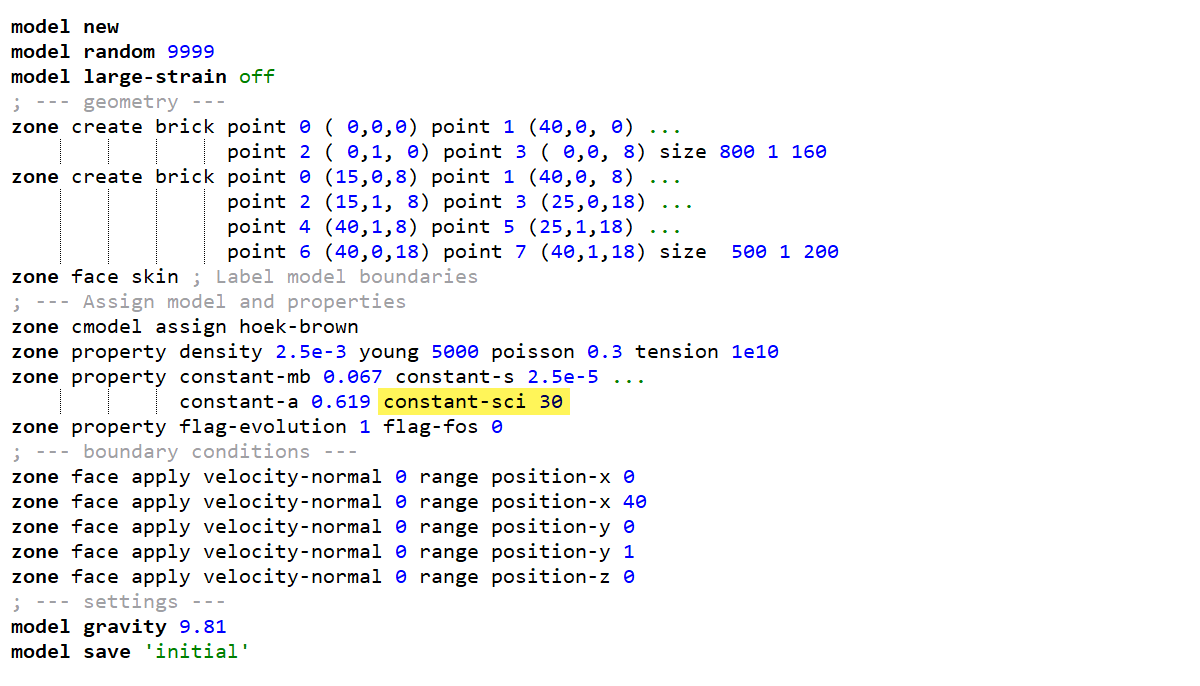In the following tutorial, the constant-sci property value in each zone will be adjusted using a pseudo-random uniform distribution of 30 ±10 MPa, which will provide a degree of rock strength variability across the model, as shown in the figure below. Of course, other properties could also be adjusted in the same manner.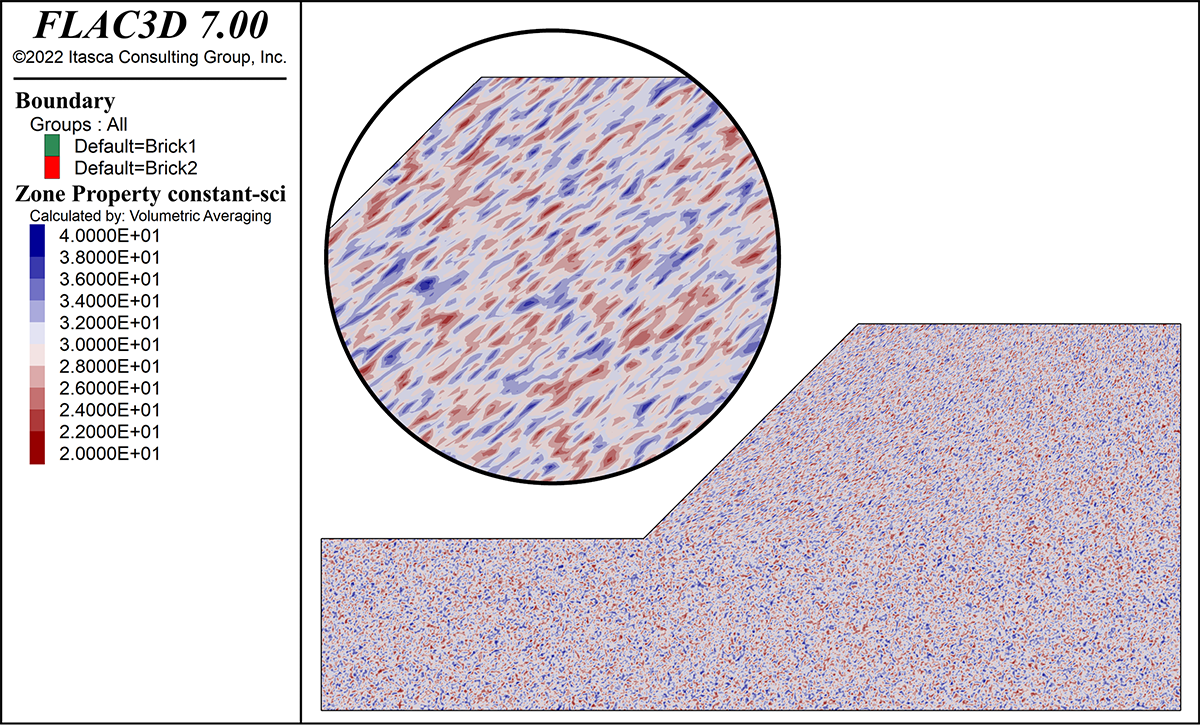Slope model showing the value of the Hoek-Brown intact rock strength property after a uniform distribution has been assigned using loops, splitting, or operators.

The following sections illustrate a series of FISH functions to demonstrate how to use loops, splitting, and operators to traverse the model zones and assign a pseudo-random distribution for the constant-sci property.

### WHILE LOOP

In the following FISH function (random_Sci_LW), the conventional While Loop is utilized. For each zone, a random value is produced using the intrinsic FISH function math.random.uniform that pseudo-randomly generates a value between 0 and 1. By multiplying this value by the lower desired strength and adding the difference between the upper and lower strengths, a distribution of values ranging from 20 to 40 MPa is produced. Properties are assigned using the intrinsic function zone.prop, where fred is the zone pointer and the property name is a string, in this case ‘constant-sci’. You can find all the property names by referencing the constitutive model in the documentation.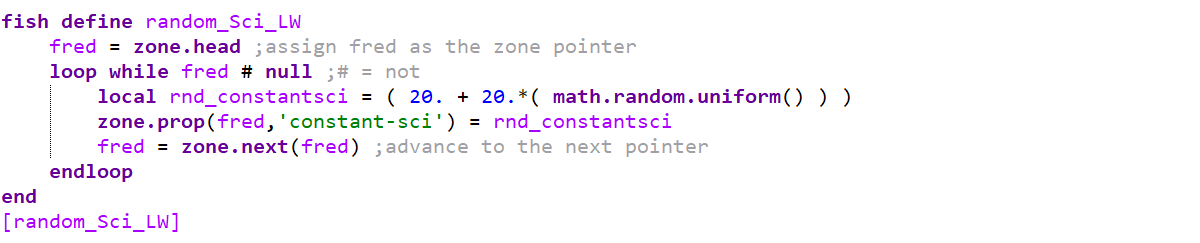### FOREACH LOOP

In the following FISH function (random_Sci_FE), the ForEach Loop is used. This syntax is more compact than the While Loop, and is available for practically every object; In the following FISH function, the loop traverses the zone.list, which contains all the zone pointers, again assigned to the variable fred. Note that the use of the local keyword before any variable is optional and provides flexibility to reuse the same variable name(s) in multiple functions without another function overriding another. Random values are generated and assigned to the constant-sci property in each zone as before.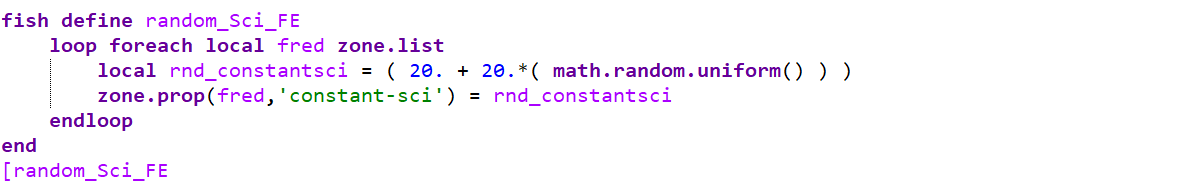### SPLITTING

Splitting can be used as an alternative to loop statements to perform actions on many objects in a very clear and concise manner. In order to make a split call, the split operator ‘::’ must prefix one or more arguments of the function, operator, or library call. Arguments that are not split will be the same in every execution of the function. As you can see in the following FISH function (random_Sci_LIST), splitting permits a very compact form with a single line of code replacing six lines in the While Loop example. On one line, zone properties randomly generated on the right-hand side of the equation are assigned to the zones in the list. Without the ::=, a single random value would be assigned to each zone constant-sci property. It's also critical that the number of zones be specified within the math.random.uniform intrinsic FISH function so that a similar number of values as there are zones are generated. This can be done easily using the intrinsic FISH function list.size.

Effectively using splitting requires a certain change of perspective and approach from traditional sequential programming. But once the user becomes comfortable, the reward is being able to very quickly and relatively efficiently perform operations on large quantities of data using a relatively small amount of code.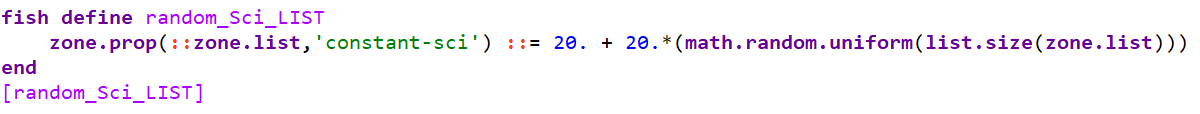### OPERATORS

While splitting is very convenient, it is, in general, not as efficient in a multi-threaded environment as a FISH operator that does multiple calculations on a single object at the same time using a single split. If speed is important (as is generally the case for functions executing during cycling, for example), it is almost always worth the effort to create an operator instead of using multiple splitting implementations on existing Intrinsics.

FISH operators are a special class of function designed to be executed in a multi-threaded environment.

Operators are created using the fish operator command, with arguments following just like a regular FISH function using the fish define command. The only difference is that there is no loop; the zone pointer is simply an input to the operator.

In the following FISH operator example (also called random_Sci_LIST), the operator is declared by specifying fish operator (rather than fish define). An input (fred) is given as an argument. The zone constant-sci property is pseudo-randomly generated and assigned as in the loop examples. However, when the operator is called, the operator input fred is defined by splitting the zone.list

On a typical modern multi-core computer and a large set of data, this approach can result in a very significant increase in speed.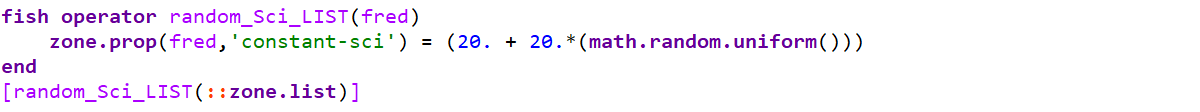### PERFORMANCE

A timing test was run five times for each of the FISH functions above by calling the time.clock intrinsic function at the start and end of the function and calculating the difference. The following figure shows the average time required for each approach discussed above (in hundredths of a second) for a model with 228,000 zones. The test was performed on an i9 CPU (3.7 GHz) with 64 GB RAM and 10 cores (20 logical processors).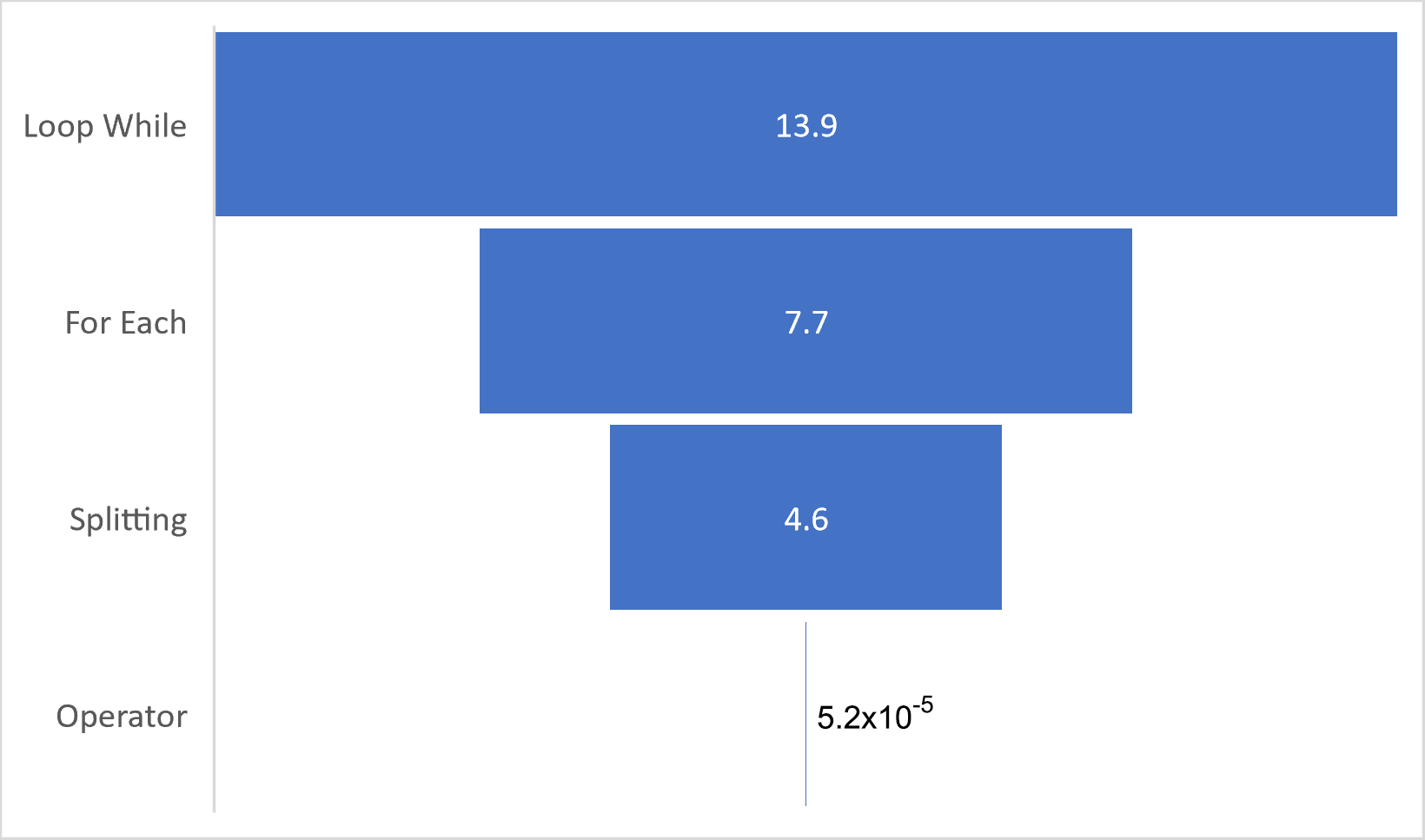Time required for each approach discussed above (in hundredths of a second) for a model with 228,000 zones.

While any of the methods reviewed above are effectively instantaneous, the performance will become more important for very large models with millions or tens of millions of objects (zones, blocks, etc.) and/or if such functions are being called during cycling (i.e., each step). As such, a method (operators) that is over 260,000 times faster than another (loop while) becomes highly desirable.

## Property-Distribution Command

Please note that all of the above FISH functions can be replaced with the zone property-distribution command and specifying a mean value of 30 MPa and a uniform standard deviation of 10 MPa:

zone property-distribution constant-sci 30 deviation-uniform 10

Of course, for this Hoek-Brown slope example, using the above command would be the best way to achieve a random uniform property distribution for constant-sci. As commands are compiled C++ code, they are ultimately going to execute faster than any function. However, the intent of this tutorial is to illustrate, hopefully in a useful way, how to employ loops, splitting, and operators — and why you might use one over another.